# Enzyme Kinetics : Kinetic Study of Enzymatic Reactions

Enzyme kinetics is the study of the chemical reactions that are catalyzed by enzymes. In enzyme kinetics, the reaction rate is measured and the effects of varying the conditions of the reaction are investigated. Studying an enzyme’s kinetics in this way can reveal the catalytic mechanism of this enzyme, its role in metabolism, how its activity is controlled, and how a drug or an agonist might inhibit the enzyme.

Enzymes are usually protein molecules that manipulate other molecules — the enzymes ‘substrates’. These target molecules bind to an enzyme’s active site and are transformed into products through a series of steps known as the Enzymatic mechanism.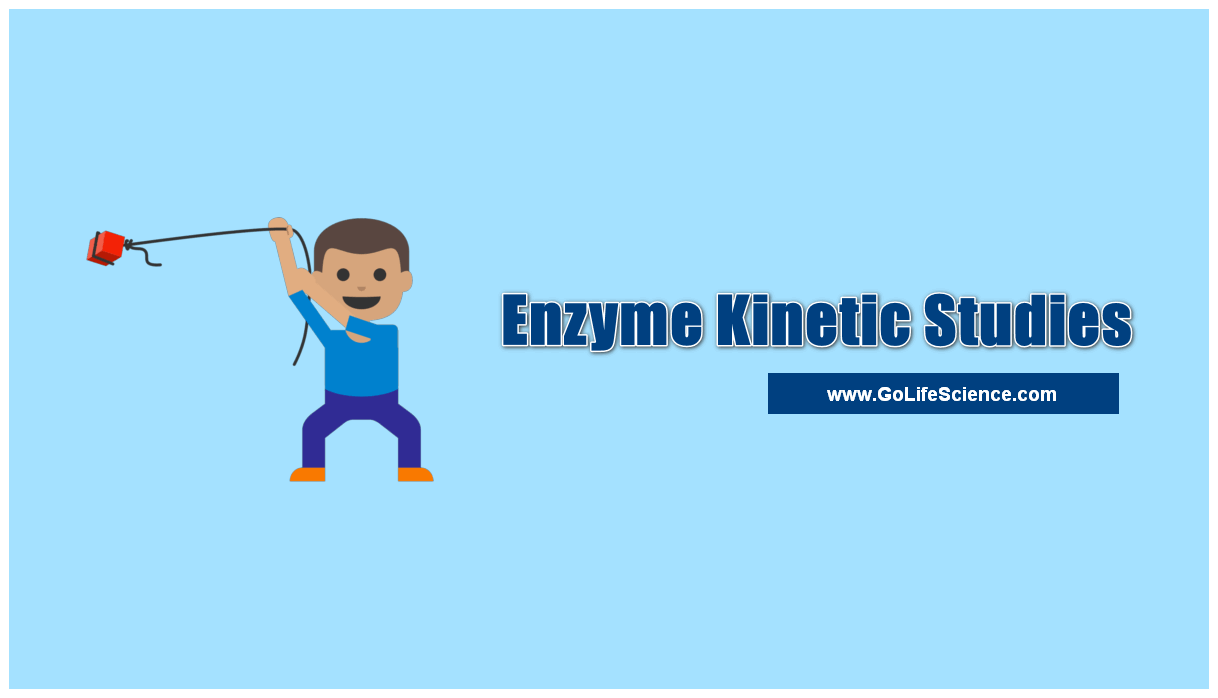These mechanisms can be divided into single-substrate and multiple-substrate mechanisms. Kinetic studies on enzymes that only bind one substrate, such as triosephosphate isomerase, aim to measure the affinity with which the enzyme binds this substrate and the turnover rate. Some other examples of enzymes are phosphofructokinase and hexokinase, both of which are important for cellular respiration (glycolysis).Enzymes with single-substrate mechanisms include isomerases such as triosephosphate isomerase or bisphosphoglycerate mutase, intramolecular lyases such as adenylate cyclase and the hammerhead ribozyme, an RNA lyase.

However, some enzymes that only have a single substrate do not fall into this category of mechanisms. Catalase is an example of this, as the enzyme reacts with the first molecule of hydrogen peroxide substrate, becomes oxidized and is then reduced by a second molecule of substrate.

Although a single substrate is involved, the existence of a modified enzyme intermediate means that the mechanism of catalase is actually a ping–pong mechanism, a type of mechanism that is discussed in the Multi-substrate reactions section below.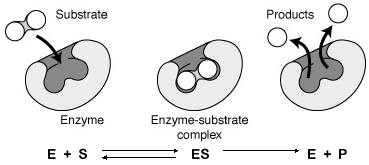Enzyme kinetics studies the speed of the reactions catalyzed by enzymes. These studies provide direct information about the mechanism of the catalytic reaction and the specificity of the enzyme. The rate of a reaction catalyzed by an enzyme can be measured relatively easily since in many cases it is not necessary to purify or isolate the enzyme.

The measurement is always made in the optimum conditions of pH, temperature, the presence of co-factors, etc., and saturating concentrations of the substrate are used. Under these conditions, the observed rate of reaction is the maximum velocity (V max). The speed can be determined by measuring the appearance of the products or the disappearance of the reactants.

To study enzyme kinetics were measured the effect of the initial concentration of substrate on the initial rate of reaction while maintaining constant the amount of enzyme. Denoting v 0 versus [S] 0 obtain a graph as that of Figure on the right. When [S] 0 is small, the initial velocity is directly proportional to the substrate concentration, and therefore, the reaction is first order. A t high [S] 0 , the enzyme is saturated with substrate, and the speed does not depend on [S] 0 . At this point, the reaction is zero order and the maximum speed (V max ).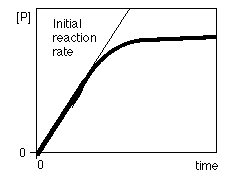To study enzyme kinetics were measured the effect of the initial concentration of substrate on the initial rate of reaction while maintaining constant the amount of enzyme. Denoting v 0 versus [S] 0 obtain a graph as that of Figure on the right. When [S] 0 is small, the initial velocity is directly proportional to the substrate concentration, and therefore, the reaction is first order. At high [S] 0 , the enzyme is saturated with substrate, and the speed does not depend on [S] 0 . At this point, the reaction is zero order and the maximum speed (V max ).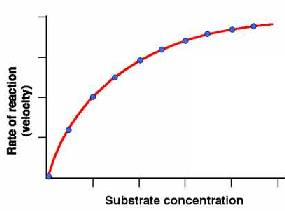## Enzyme Kinetics – Michaelis–Menten kinetics

In 1913, Linor Michaelis  (1875-1949) and Maud Menten (1879-1960) put forward the enzyme-substrate complex theory. According, the enzyme (E) combines with the substrate(S), to form an enzyme-substrate(ES) complex, which immediately breaks down to the Enzyme and the Product (P).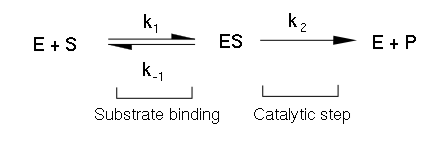The above reactions are assumed to be reversible. Here k1, k2, k3, k4 are specific rate constants. Michelis-Menton equation is the rate equation for the reaction catalyzed by an enzyme having a single substrate. In this derivation that the Brigg’s and Halden.

• Molar Concentration of [E] =Concentration of free (or) free (or) uncombined enzyme
• [ES]=Concentration of  Enzyme-Substrate complex
• [Et]=Total enzyme concentration (the sum of the free and combined forms)
• [S]=Concentration of Substrate
• [P]=Concentration of Product

The substrate concentration is assumed to be far greater than the concentration of Enzyme [E]. So that the amount of substrate bound by the enzyme at any given time is negligible. Compared with the total concentration of [S].

Systematic studies of the effect of substrate concentration on ionic enzyme activity began performing in the late nineteenth century. Already in 1882, the concept of an enzyme-substrate complex as an intermediary in the process of enzymatic catalysis was introduced. In 1913, Leonor Michaelis (pictured left) and Maud Menten (pictured right) developed this theory and proposed a rate equation that explains the kinetic behavior of the enzyme.

To explain the observed relation between the initial velocity (v0) and the initial substrate concentration ([S]0) Michaelis and Menten proposed that the enzymatically catalyzed reactions occur in two phases :

• The first phase: In the first stepthe enzyme-substrate complex is formed.
• Second Phase: The enzyme-substrate complex results in the formation of the product, releasing the free enzyme.

In this scheme, k 1 , k 2 and k 3 are constants each individual kinetics of the process and also are called microscopic rate constants . Accordingly, we can say that:

• v 1 = k 1 [E] [S]
• v 2 = k 2 [ES]
• v 3 = k 3 [ES]

One can distinguish between free enzyme (E) and attached to the enzyme substrate (S), so that the total concentration of enzyme , [E T ], (which is constant throughout the reaction) is:

[E T ] = [E] + [ES]

As [E] = [ET] – [ES], it follows that:

v 1 = k 1 [S] [E T ] – k 1 [S] [ES]

This kinetic model adopts the steady-state hypothesis , according to which the concentration of the enzyme-substrate complex is small and constant throughout the reaction (Figure, right). Therefore, the rate of formation of the enzyme-substrate complex (v 1 ) is equal to that of its dissociation (v 2 + v 3 ):

v 1 = v 2 + v 3

Further, as [ES] is constant, the rate of formation of the products is constant:

v = v 3 = k 3 [ES] = constant .

As v 1 = v 2 + v 3 , we can say that:

k 1 [S] [E T ] – k 1 [S] [ES] = k 2 [ES] + k 3 [ES]

Solving for [ES], is that: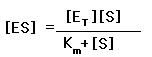being km=(k2+k3) / k1, where the expression (k 2 + k 3 ) / k 1 has been replaced by K M , or Michaelis-Menten constant . This link gives us an explanation of the reasons that make the K M an important kinetic parameter .

Thus, at steady state, the rate of formation of the product is:

v = v 3 = k 3 [ES] =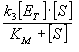For any enzyme, [E reaction T], k 3 and KM are constants. Let us consider two extreme cases:

• A small substrate concentrations ([S] << KM ) = v (k3[ET] /KM) [S]. As the terms in parentheses are constant, they can be included in a new constant, k obs , so that the expression is reduced to: v = k obs [S] , so that the reaction is a first order kinetic process .
• A high substrate concentrations ([S] >> KM), v = k 3 [E T ] . The reaction rate is independent of the concentration of the substrate, and therefore, the reaction is zero order kinetic process . In addition, both k 3 and [E T ] are constant, and allows us to define a new parameter, the maximum reaction rate (V max ): V max = k 3 [E T ], which is the rate that would be achieved when all available enzyme bound to the substrate is at.

If we introduce the parameter V max in the general rate equation (boxed formula above), we obtain the best known of the Michaelis-Menten expression :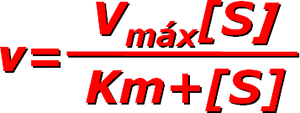There are enzymes that do not obey the Michaelis-Menten equation. It says its not Michaelian kinetics. This happens with Allosteric enzymes , whose graph v vs. [S] is not hyperbole, but a sigmoid (figure right). The Sigmoidal kinetics , small variations in [S] in a critical area (near K M ) results in large variations in the reaction rate.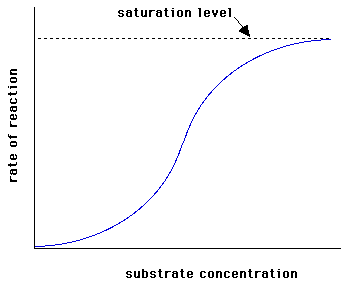## Enzyme Kinetics: Calculation of Km and Vmax

The graphical representation of the Michaelis-Menten equation (v 0 versus [S]0 ) is a hyperbola (figure left). The V max is the maximum value that tends the experimental curve and the KM corresponding to the substrate concentration at which the reaction rate is half of the V max.  To graphically determine the values of Km and Vmax is easier to use the dual representation reciprocal (1/V0 versus 1/[S]0), since it is a straight line. This reciprocal dual representation is called Lineweaver-Burk. It is a line in which:

• The slope is Km/Vmax
• The abscissa at the origin (1/V0 = 0) is -1 /Km
• The intercept (1/[S] 0 = 0) is 1/Vmax

Thus, from the experimental data can be calculated graphically, the values of K M and V max of an enzyme for different substrates.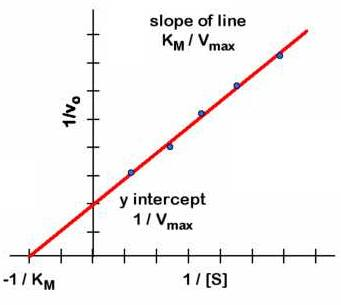## Enzyme Activity

Defines the enzyme unit (U) as the quantity of enzyme that catalyzes the conversion of 1 mol of the substrate in one minute. The specific activity is the number of units of enzyme per milligram of protein (U / mg port) or per milliliter of solution (U / ml).

Recently, the International System of Units (SI) has defined the unit of enzyme activity as the amount of enzyme that converts 1 mol of substrate per second. This unit is called katal (kat). As 1 mole is 106 pmol and 1 minute is 60 seconds then 1 katal is equivalent to 60 x 10 6 U. This unit is very large, so that as the micro-katal sub-multiples (ĩkat, 10 are commonly used -6 kat) or nano-katal (nkat 10 -9 kat).

When the molecular weight of pure enzyme and the number of active sites per enzyme molecule is known, measurements of enzyme activity to calculate the turnover number of the enzyme, that is, the number of elementary reactions that makes the enzyme active site per and per unit time.

## Statement-making of Km an Important Kinetic Parameter

The Michaelis-Menten constant (Km) is an important kinetic parameter for multiple reasons: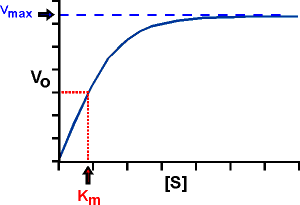• M is the concentration of substrate to which the reaction rate is half of the maximum speed. Indeed, if K M = [S], the Michaelis-Menten equation reduces to: v = V max / 2.
• The value of Ksuggests the affinity of the enzyme for the substrate: A lower K M , higher affinity of the enzyme for the substrate, and the higher K M , lower affinity. This fact is easily explained if we consider that KM is defined as (k2+ k3 / k1), where the reactions 2 and 3 destroy the ES complex, while reaction 1 is formed. Thus, if KM is large, the ES complex is unstable because the prevailing tendency to destroy (low affinity toward the substrate), and if KM is small, the ES complex is stable as predominant tendency to form it (high affinity toward the substrate).
• The KM of the natural substrate is less than that of analogous substrates. If two same enzyme substrates have different K M , which present higher KM has a lower affinity for the enzyme, and the reaction always takes place more slowly than with the substrate lower KM , except at saturating substrate concentrations, where v = Vmax .
• The values of KM of many enzymes are close to the physiological concentration of the substrates, so that small variations in the [S] may represent large changes in the speed of the whole metabolic pathway.

## Summary

This chapter Enzyme kinetics study explained the basics of enzyme kinetic equation, Enzyme activity, Michaelis-Menton equation, and Km parameter importance.